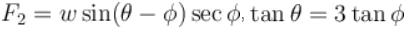Courses

# Friction Force – MSQ

## 10 Questions MCQ Test Basic Physics for IIT JAM | Friction Force – MSQ

Description
This mock test of Friction Force – MSQ for Physics helps you for every Physics entrance exam. This contains 10 Multiple Choice Questions for Physics Friction Force – MSQ (mcq) to study with solutions a complete question bank. The solved questions answers in this Friction Force – MSQ quiz give you a good mix of easy questions and tough questions. Physics students definitely take this Friction Force – MSQ exercise for a better result in the exam. You can find other Friction Force – MSQ extra questions, long questions & short questions for Physics on EduRev as well by searching above.
*Multiple options can be correct
QUESTION: 1

### Two block of masses m1 and m2 are connected with a massless unstretched spring and placed over a plank moving with an acceleration a as shown in figure. The coefficient of friction between the blocks and platform is µ. Choose the correct statements :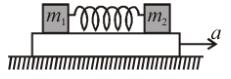Solution:

Let the value of a be increased from zero. As long as a ≤ μg  there shall be no relative motion between m1 or m2 and platform, that is, m1 and m2 shall move with acceleration a.
As a > µg the acceleration of m1 and m2 shall become µg each.
Hence at all instant the velocity of m1 and m2 shall be same
∴ The spring shall always remain in natural length.

The correct answers are: spring will be stretched if a < μg , spring will be compressed if a ≤ μg , spring will neither be compressed nor be stretched for a ≤ μg

*Multiple options can be correct
QUESTION: 2

### All the blocks shown in the figure are at rest. The fully is smooth and the strings are light. Coefficient of friction at all the contacts is 0.2. A frictional force of 10 N acts between A and B. The block A is about to slide on block B. The normal reaction and frictional force exerted by the ground on the block B is.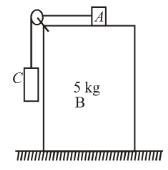Select one or more:

Solution:

The friction force on block A is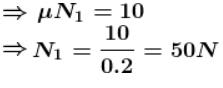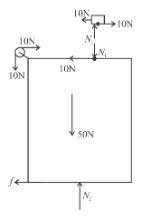The net force on block B in vertical direction is zero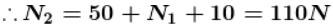⇒ Normal reaction exerted by ground on block B is 110 N.
frictional force exerted by ground on block B is zero

The correct answers are: The normal reaction exerted by the ground on the block B is 110N, The frictional force exerted by the ground on the block B is zero

*Multiple options can be correct
QUESTION: 3

### A block A (5 kg) rests over another block B (3 kg) placed over a smooth horizontal surface. There is friction between A and B. A horizontal force F1 gradually increasing from zero to a maximum is applied to A so that the blocks move together without relative motion. Instead of this another horizontal force F2, gradually increasing from zero to a maximum is applied to B  so that the blocks move together without relative motion. Then :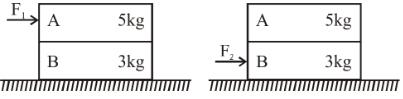Select one or more:

Solution: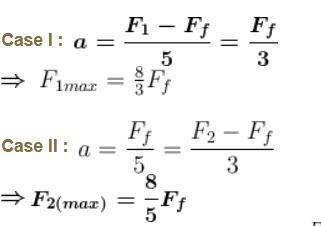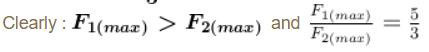The correct answers are: F1(max) > F2 (max), F1(max) : F2 (max) = 5 : 3

*Multiple options can be correct
QUESTION: 4

Two blocks of masses 5kg and 3kg are placed in contact over an inclined surface of angle 37°, as shown. µ1 is friction coefficient between 5kg block and the surface of the incline and similarly, µ2 is friction coefficient between the 3kg block and the surface of the incline. After the release of the blocks from the inclined surface,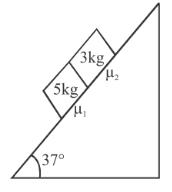Select one or more:

Solution:

Case-I : μ1 = 0.5, μ2 = 0.3
Along the incline, acceleration of 5kg block will be less than acceleration of 3kg block provided they move alone on the incline. The reason is greater friction coefficient of 5kg block, as acceleration along the incline is g sin θ - μg cos θ
Hence, both blocks will move together. In this case FBDs of both are shown.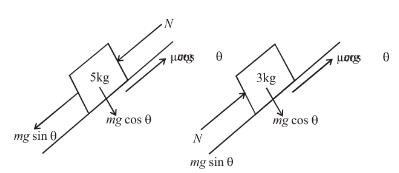For 5 kg block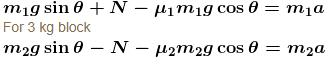Case II :  acceleration of 5 kg block is more than acceleration of 3 kg. Hence, no normal force will act between them.

The correct answers are: if µ1 = 0.5 and µ2 = 0.3 then 5kg block exerts 3N force on the 3kg block, if µ1 = 0.3 and µ2 = 0.5 then 5kg block exerts no force on the 3kg block

*Multiple options can be correct
QUESTION: 5

The value of mass m for which the 100 kg block remains in static equilibrium is :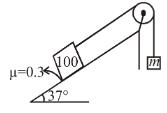Select one or more:

Solution: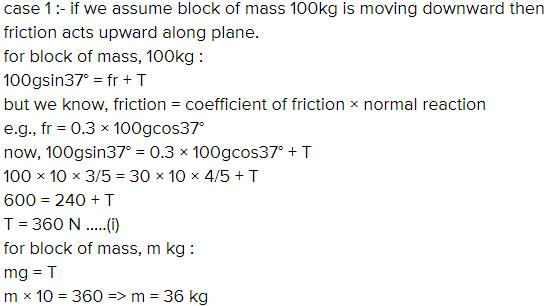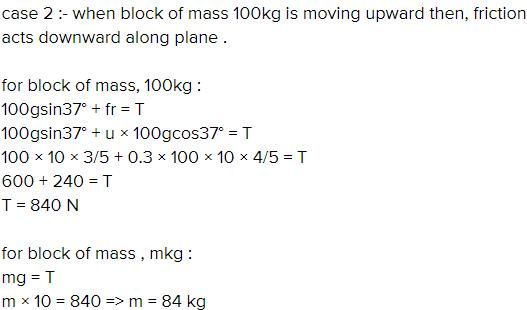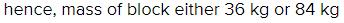*Multiple options can be correct
QUESTION: 6

A block of mass 1kg is stationary with respect to a conveyor belt that is acceleration with 1m/s2 upwards at an angle of 30° as shown in figure. Which of the following is/are correct?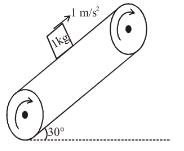Select one or more:

Solution:

Block is moving upwards due to friction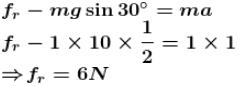Contact force is the result of N and fr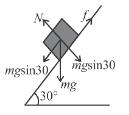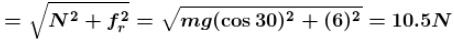The correct answers are: Force of friction on block is 6N  upwards, Contact force between the block and belt is 10.5N

*Multiple options can be correct
QUESTION: 7

A plank of mass m1 = 8kg  with a bar of mass m2 = 2kg  placed on its rough surface, lie on a smooth floor of elevator ascending with an acceleration g/4. The coefficient of friction is µ = 1/5 between is m1 and m2. A horizontal force F = 30N is applied to the plank. Then choose the correct statements :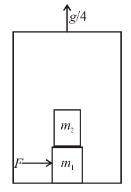Select one or more:

Solution:

FBD in reference frame of the lift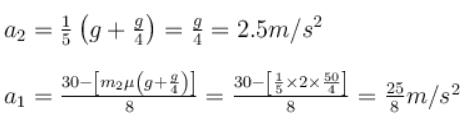The correct answers are: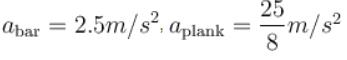*Multiple options can be correct
QUESTION: 8

In the shown arrangement, if f1f2 and T be the frictional forces on 2kg block, 3kg block and tension in the string respectively, choose the correct statements :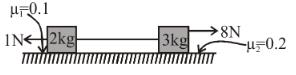Select one or more:

Solution:

FBD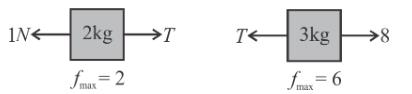Net force without friction on system is ‘7N’ in right side so first maximum friction will come on 3 kg block.
So, F1 = 1 NF2 = 6NT = 2 N.
The correct answers are: f1 = 1N , f2 = 6NT = 2N

*Multiple options can be correct
QUESTION: 9

Two blocks with masses M1 and M2 of 10 kg and 20 kg respectively are placed as in figure. Coefficient of friction µ = 0.2 between all surfaces, then choose the correct statement.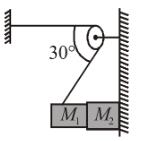Select one or more:

Solution:

Since block M1 is attached to the string so it will remain stationary. M2 has tendency to move downward.
So, friction on M2 will act in upward direction.
N is the force by which the both block are pressed i.e. N = Tcos 30°.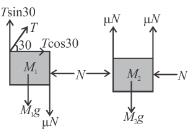for block M1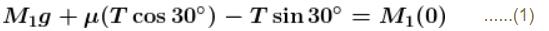T = 306.75N
T ≈ 306 N
(a = 0 for M1 since attach to string)
for block M2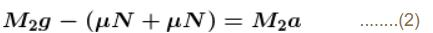a = 4.67ms–2
The correct answers are: Tension is 306 NaM2 = 4.7 m/s2

*Multiple options can be correct
QUESTION: 10

The force F1 that is necessary to move a body up an inclined plane is double the force F2 that is necessary to just prevent it from sliding down then :

Select one or more:

Solution: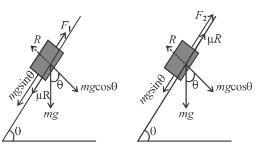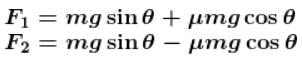But mg = w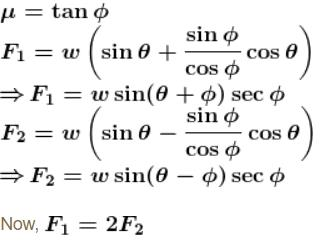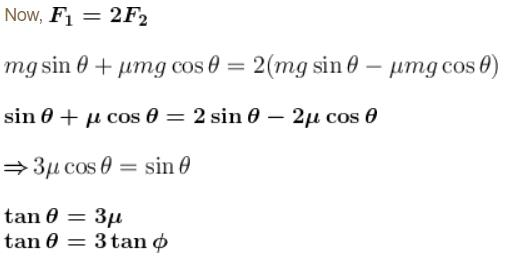The correct answers are: